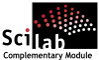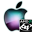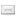ATOMS : Condition Number details

# Condition Number

Evaluates the condition number of functions.Details
Version
0.2
A more recent valid version exists: 0.2.2
Author
Michael Baudin
Owner Organization
DIGITEO
Maintainer
Michael BAUDIN
Category
Dependency
Creation Date
February 3, 2011
Source created on
Scilab 5.2.x
Binaries available on
Scilab 5.2.x:Windows 64-bitWindows 32-bitLinux 64-bitLinux 32-bitmacOS
Install command
`--> atomsInstall("condnb")`
Report a bugDescription
```            Purpose
----

These functions provides the condition number of various elementary functions.
Their goal is to evaluate the potential numerical difficulty of evaluating
numerically a function at a given point.
We may use this module while testing a given function.

The toolbox is based on macros.

To get an overview of the functions in this module, use :

help condnb_overview

This module uses the apifun module.

Features
--------

The following is a list of the current functions :
* condnb_condnum : Computes the empirical condition number of the function f at
point x.
* condnb_acoscond : Computes the condition number of the acos function.
* condnb_asincond : Computes the condition number of the asin function.
* condnb_atancond : Computes the condition number of the atan function.
* condnb_coscond : Computes the condition number of the cos function.
* condnb_erfcond : Computes the condition number of the erf function.
* condnb_erfinvcond : Computes the condition number of the erfinv function.
* condnb_expcond : Computes the condition number of the exp function.
* condnb_logcond : Computes the condition number of the log function.
* condnb_plotcond : Plots the condition number of a function.
* condnb_powcond : Computes the condition number of the pow function.
* condnb_sincond : Computes the condition number of the sin function.
* condnb_sqrtcond : Computes the condition number of the square root function.
* condnb_sumcond : Computes the condition number of the sum function.
* condnb_tancond : Computes the condition number of the tan function.

Forge
-----

http://forge.scilab.org/index.php/p/condnb/
```Files (2)Source code archive
```
```OS-independent binary for Scilab 5.2.x
```Binary version
Automatically generated by the ATOMS compilation chain

```News (0)Comments (2)Comment -- February 3, 2011, 05:30:05 PM
```Changes for version 0.1:

condnb (0.1)
* Created module from functions in the assert module.
* Added unit tests for exp, log, pow and sqrt.
* Updated Overview ; added Quick Start.
* Updated implementation of plotcond.
* Use apifun.
```Comment -- February 3, 2011, 05:30:19 PM
```Changes for version 0.2:

condnb (0.2)
* Fixed ticket #260: the plot example in acos did not work.
```Leave a comment
Login withEmail notifications